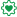# New to Qlik Sense

Discussion board where members can get started with Qlik Sense.

Announcements
BI & Data Trends 2021. Discover the top 10 trends emerging in today. Join us on Dec. 8th REGISTER
cancel
Showing results for
Did you mean:
HighlightedContributor II

## How to create new Variable based on another multiple Variable using if, else statement ??

Hi, I am looking for any advice on how to create variable and call in Qlik Sense report.

Q. How to create a new variable using (filter, if, if else statement) on a different variable on the same calculated field? Please find the attached document of this power bi DAX Queries? Is the syntax similar in Qlik Sense or any option?

Power BI DAX Queries given below. I need syntax similar to these:-

 Column1 Column2 Column3 Column4 Column5 Permission.Check 1 Permission John Allow Permission 1 Permission John Allow Permission 1 Delete John Allow Permission 2 Permission John Allow Delete 2 Permission John Allow Delete 2 Delete John Allow Delete 2 Delete John Allow Delete 3 Permission John Allow 3 Delete John Allow 4 Permission John XYZ Allow 4 Delete John Deny

 Permissions.Check =VAR Permissions = CALCULATE(COUNTROWS(TableName),ALLEXCEPT(TableName,TableName[column1],tableName[column2]))VAR Delete =    CALCULATE(        COUNTROWS(TableName),            FILTER(                ALLEXCEPT(TableName,TableName[column1],TableName[column2]),                    TableName[column3]="John"&&                    ISBLANK(TableName[column4])                )            )RETURNSWITCH(TRUE(),    TableName[column5]<>"Allow",BLANK(),    Permissions=2&&Delete=1,"Permission",    Permissions=2&&Delete=2,"Delete",   BLANK())
Labels (2)

• ### Qlik Sense

9 Replies
HighlightedSpecialist II

You can find  a icon (x=) embed on the bottom left of the screen , click on that to create and define variables once a variable is defined you can call it in the expression window using \$ Symbole like \$(var_name)

https://help.qlik.com/en-US/sense/November2019/Subsystems/Hub/Content/Sense_Hub/Variables/create-var...

HighlightedEmployee

I am not familiar with Power BI DAX Queries, so I don't understand what you are trying to do. Could you please describe the logic of what you are trying to achieve?

Further, I don't understand if you want to add a calculated column in the data model, or if you want to do it in a table in the UI. I assume that it is in the UI, but you don't mention anything about dimensions in your table, so I am not sure.

Anyway, if it is in the data model, you should just add a field in the Load statement in the script. But if it is in the UI, you need to create a measure in the object.

In both cases, you need to have an expression like If(<Condition>,'Permission','Delete') to define the content of the column, and here you can see where you need to define your logic.

Finally, in the UI the condition needs to use aggregation functions, otherwise it will not always be defined.

HighlightedContributor II

Hi Henric,

Can you provide the example of aggregation functions that you have written above?

HighlightedEmployee
HighlightedMVP

I am trying to help your colleague may be with the same problem, but I don't really understand this well enough to answer

HighlightedContributor II

Hello Sunny

We have created Variable in Power Bi which is coming up in Columns, and here what we are looking for is that We need to create a Dimension which end up showing the result in the column.Also is it possible to create the Dimension through Variable ?
I believe you might have understood my concern now. Kindly help for the same.

HighlightedEmployee

You can do a lot with a Qlik variable, but don't let the name "variable" fool you. In the Qlik environment there is probably another, better way to do it: Perhaps you should define a master measure.

Do the following:

1) Figure out whether you want this new column in the data model (on the lowest, atomic level of data) or in the UI (aggregated numbers, recalculated based on the user selection)
2) Figure out what the expression should be. Test it to make sure that it does what you want.
3) Figure out how you can make this expression re-usable. Probably you should define a master measure. (But perhaps a master dimension is a better option? Or you can put it in a variable that is expanded to the wanted expression, whenever the variable is used.)

Good luck

HIC

HighlightedContributor II

Now it Solved!

Now I have a question regarding dimension.

Q. We have created dimensions, And we needed to create a count value in Dimension which ends up showing the result in the column (Table Chart).

Thank You!

HighlightedPartner

Greetings,

Perhaps it might be too late for your purposes, but, since I have never used DAX, it seemed like an interesting challenge to reverse engineer your DAX calculation to try to understand what you wanted to achieve, and then try to find the equivalent Qlik expression.

I  believe the following expression solves your problem:

if(
Column5 <> 'Allow', '',
if(
aggr(NODISTINCT count({\$<Column1=,Column2={'Permission'}>} Column1), Column1) = 2 // Permissions
and
aggr(NODISTINCT count({\$<Column1=,Column2={'Delete'},Column3={'John'},Column4={''}>}Column1), Column1) = 1, // Delete
'Permission',
if(
aggr(NODISTINCT count({\$<Column1=,Column2={'Permission'}>} Column1), Column1) = 2 // Permissions
and
aggr(NODISTINCT count({\$<Column1=,Column2={'Delete'},Column3={'John'},Column4={''}>} Column1), Column1) = 2, 'Delete',
''
)))

You just need to use it as the metric of a Qlik Sense table.  If you want to reuse the Permission and Delete macros in other expressions, then all you need to do is:

1. Store aggr(NODISTINCT count({\$<Column1=,Column2={'Permission'}>} Column1), Column1) in a variable called Permission and do the same for Delete using as its value aggr(NODISTINCT count({\$<Column1=,Column2={'Delete'},Column3={'John'},Column4={''}>}Column1), Column1); and,
2. Invoke these variables as if they were macros wherever you need them using \$ sign expansion as in \$(Permission) and \$(Delete).

From what I've read about the ALLEXCEPT function in DAX, the equivalent functionality is achieved by a combination of using the \$ sign in a set analysis expression and then explicitly indicating the fields for which you want to ignore any user applied filters, such as in Column1= above.

There is no SWITCH conditional function in Qlik either, so I used nested IFs.  Sometimes, depending on the situation, people use a combination of PICK(MATCH(expression, value, vale,,,value), expression, expression,,,expression) to simulate a SWITCH.

Cheers,

++José